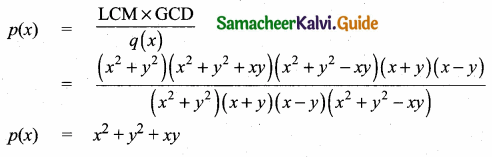Students can download Maths Chapter 3 Algebra Ex 3.3 Questions and Answers, Notes, Samacheer Kalvi 10th Maths Guide Pdf helps you to revise the complete Tamilnadu State Board New Syllabus, helps students complete homework assignments and to score high marks in board exams.

## Tamilnadu Samacheer Kalvi 10th Maths Solutions Chapter 3 Algebra Ex 3.3

Question 1.
Find the LCM and GCD for the following and verify that f(x) × g(x) = LCM × GCD

(i) 21x2y, 35xy2
p(x) = 21 x2y = 3 × 7 × x2 × y
g(x) = 35xy2 = 5 × 7 × x × y2
G.C.D = 7 xy
L.C.M = 3 × 5 × 7 x2 × y2
= 105 x2y2
L.C.M × G.C.D = 105x2y2 × 7xy
= 735 x3y3 ….(1)
p(x) × g(x) = 21x2y × 35xy2
= 735x3y3 ….(2)
From (1) and (2) we get
L.C.M × G.C.D. = p(x) × g(x)(ii) (x3 – 1)(x + 1),(x3 + 1)
p(x) = (x3 – 1) (x + 1) = (x – 1) (x2 + x + 1) (x + 1)
g(x) = x3 + 1 = (x + 1) (x2 – x + 1)
G.C.D = (x + 1)
L.C.M = (x + 1) (x – 1) (x2 + x + 1) (x2 – x + 1)
L.C.M × G.C.D = (x + 1) (x – 1)(x2 + x + 1)(x2 – x + 1)x(x + 1)
= (x + 1)2 (x – 1) (x2 + x + 1) (x2 – x + 1) ……….(1)
p(x) × g(x) = (x – 1) (x2 + x + 1) (x + 1) (x + 1) (x2 – x + 1)
= (x + 1)2 (x – 1) (x2 + x + 1) (x2 – x + 1) ……….(2)
From (1) and (2) we get
L.C.M × G.C.D. = p(x) × g(x)

(iii) (x2y + xy2), (x2 + xy)
p(x) = x2y + xy2 = xy(x + y)
g(x) = x2 + xy = x(x + y)
G.C.D = x(x+y)
L.C.M = xy (x +y).
L.C.M × G.C.D = xy(x + y) × x(x + y)
= x2y(x + y)2 …..(1)
p(x) × g(x) = xy(x + y) × x(x + y)
= x2y(x + y)2
From (1) and (2) we get
L.C.M × G.C.D. = p(x) × g(x)Question 2.
Find the LCM of each pair of the following polynomials
(i) a2 + 4a – 12, a2 – 5a + 6 whose GCD is a – 2
p(x) = a2 + 4a – 12
= a2 + 6a – 2a – 12
= a (a + 6) – 2(a + 6)
= (a + 6) (a – 2)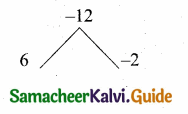g(x) = a2 – 5a + 6
= a2 – 3a – 2a + 6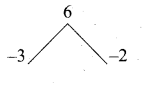= a(a – 3) – 2 (a – 3)
= (a – 3) (a – 2)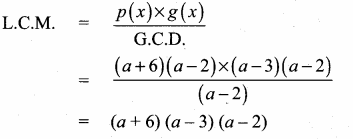(ii) x4 – 27a3x, (x – 3a)2 whose GCD is (x – 3a)
p(x) = x4 – 27a3x = x[x3 – 27a3]
= x[x3 – (3a)3]
= x(x – 3a) (x2 + 3ax + 9a2)
g(x) = (x – 3a)2
G.C.D. = x – 3a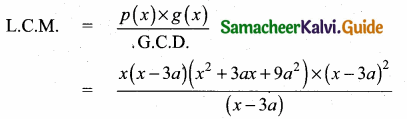L.C.M. = x (x – 3a)2 (x2 + 3ax + 9a2)Question 3.
Find the GCD of each pair of the following polynomials
(i) 12(x4 – x3), 8(x4 – 3x3 + 2x2) whose LCM is 24x3 (x – 1)(x – 2)
p(x) = 12(x4 – x3)
= 12x3(x- 1)
g(x) = 8(x4 – 3x3 + 2x2)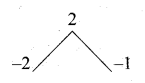= 8x2(x2 – 3x + 2)
= 8x2(x – 2)(x – 1)
L.C.M. = 24x3 (x – 1) (x – 2)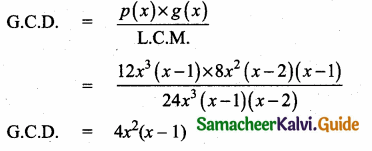(ii) (x3 + y3), (x4 + x2y2 + y4) whose LCM is (x3 + y3) (x2 + xy + y2)
p(x) = x3 + y3
= (x + y)(x2 – xy + y2)
g(x) = x4 + x2y2 + y4 = [x2 + y2]2 – (xy)2
= (x2 + y2 + xy) (x2 + y2 – xy)
L.C.M. = (x3 + y3) (x2 + xy + y2)
(x + y) (x2 – xy + y2) (x2 + xy + y2)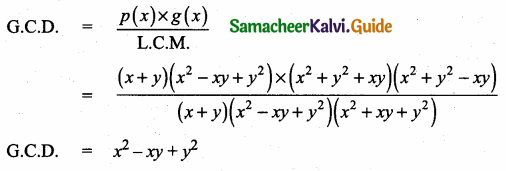G.C.D. = x2 – xy + y2Question 4.
Given the L.C.M and G.C.D of the two polynomials p(x) and q(x) find the unknown polynomial in the following table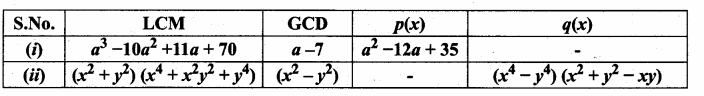L.C.M. = a3 – 10a2 + 11a + 70
= (a – 7) (a2 – 3a – 10)
= (a – 7) (a – 5) (a + 2)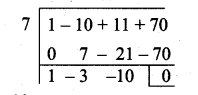G.C.D. = (a – 7)
p(x) = a2 -12a + 35
= (a – 5)(a – 7)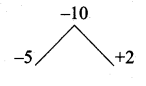q(x) = $$\frac{\mathrm{LCM} \times \mathrm{GCD}}{p(x)}$$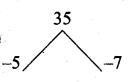(ii) L.C.M (x2 + y2)(x4 + x2y2 + y4)
(x2 + y2)[(x2 + y2)2-(xy)2]
(x2 + y2) (x2 + y2 + xy) (x2 + y2 – xy)
G.C.D. = x2 – y2
(x + y)(x – y)
q(x) = (x4 – y4) (x2 + y2 – xy)
= [(x2)2 – (y2)2](x2 + y2 – xy)
= (x2 + y2) (x2 – y2) (x2 + y2 – xy)
(x2 + y2) (x + y) (x – y) (x2 + y2 – xy)
P(x) = x2 + y2 + xy